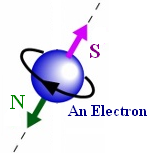## Wednesday, April 07, 2021 ...//### FNAL: Combined muon $$g-2$$ discrepancy grows to 4.2 sigma

Particles have a quantized spin (their own angular momentum) and leptons such as electrons and muons always have $$J_z = \pm \hbar /2$$. Yes, the electrons are spinning and it is other ideas than that which you need to modify (you need to switch from classical physics to quantum mechanics).

They also carry an electric charge, $$-e$$, which is effectively orbiting around the center of mass at the radius comparable a "typical dimension of the particle" (which is inversely proportional to the particle mass) and therefore creates an electric current.So the particles also behave just like solenoids and they are also magnets with a magnetic moment. Adopting Feynman's pragmatic definition that I completely sympathize with, the magnetic moment $$\vec \mu$$ is a vector that multiplies the magnetic field $$-\vec B$$ in a term in the Hamiltonian, $\Delta H = -\vec \mu \cdot \vec B.$ How big is the electron magnetic moment? Well, it is (if I choose one form)$\vec \mu_s = -g_s \frac{e}{2m_e} \cdot \vec S$ where $$g_s\approx 2$$. Note that the Bohr magneton $$\mu_B$$ is sometimes used which represents the constant $$e\hbar / 2 m_e$$ in the SI units. The Dirac equation gives you $$g_s=2$$ exactly.

Famously enough, Quantum Electrodynamics (QED) teaches us that the Dirac equation's answer $$g_s=2$$ isn't quite accurate. It is just a "classical approximation" in the sense of classical fields and there are additional "quantum corrections" which are encoded in loop (Feynman) diagrams. The simplest one involves the radiation and re-absorption of one photon (a "triangle diagram" corresponding to a 1948 calculation by Julian Schwinger) but there exist diagrams of an arbitrarily high complexity (or the number of loops) whose contribution decreases as the number of loops goes up.

The most precise refinement of the number 2 is a value measured in 2006 by Gerry Gabrielse and pals at Harvard,$g_s \approx 2.00231930436182(52).$ We are almost certain about 13 significant digits; it is arguably still the most precise successfully verified prediction in natural sciences (only "$$2+2=5$$ because of racism", a great recent insight made in the humanities, is more safely and precisely validated). And to make things amazing, the value may also be calculated theoretically and these 13 digits agree! Note that the aforementioned subleading approximation due to Schwinger is$g_s \approx 2 \zav { 1+\frac{\alpha}{2\pi} }$ where $$\alpha\approx 1/137.036$$ is the fine-structure constant. Fine. You may repeat the same story for the muon. Its magnetic moment will be about 206.8 times smaller because the muon is 206.8 times heavier than the electron but it will be expressed by a nearly identical formula, with the muon mass, and even the subleading correction from the fine-structure constant will be exactly the same. The even lower corrections will be different, however, and the precision won't be quite as amazing as one for the electron.

Today, the Fermilab announced a new result:

First results from the Muon $$g-2$$ experiment at Fermilab
‘Last Hope’ Experiment Finds Evidence for Unknown Particles (Quanta Magazine, Natalie Wolchover)
New Muon $$G-2$$ Results! (Tommaso Dorigo's response written on behalf of the world's sourballs)
Check also Jester but that one is an April Fools' Joke and if I didn't tell you explicitly, 99% of the readers wouldn't get it.

The theoretical and experimental values deviate. The deviation is 3.3 sigma (about 99.9% formal certainty of a new effect) from the new result only and 4.2 sigma (over 99.999% certainty, formally) where sigma is the standard deviation. That is pretty exciting, short of 5 sigma, however, but it seems convincing that it's not a pure statistical fluke. Something is going on. Either the experiment has some systematic error (e.g. a cable was incorrectly made of a chewing gum); or the theory has it (or both).

The theoretical systematic error may be due to new particles running in the loops (beyond the Standard Model) which would be exciting and almost any model of new physics around the corner predicts such corrections to the muon $$g-2$$; or the theoretical error may be due to some unjustified approximation that is believed to be justified, probably related to the mixing of the muon with hadrons or to the instability of the muon, I would personally add.

Wolchover's title involving the "last hope" is dramatic, as expected from the people that the Czech president Zeman called "hyenas" again today. ;-) But it is a misleading phrase, indeed. No "hope for new physics" can ever be "the last one" because there cannot be any "end of science". Every theory or claim, especially a messy and clearly incomplete formula for a muon $$g-2$$, must always be considered provisional in science. Everyone who denies this basic claim about any fudged up formula or theory is denying the rudiments of science.

We may need a lot of time to find out what is actually the reason for this discrepancy. Note that when it comes to the LHC anomalies, we recently noticed a 2.8-sigma deviation supporting stealthy supersymmetry and new claims by the LHCb that they observe a violation of the lepton universality (check Googlew News; I didn't write a new article because it wouldn't be sufficiently new but this anomaly may be the most convincing among these three).#### snail feedback (0) :

(function(i,s,o,g,r,a,m){i['GoogleAnalyticsObject']=r;i[r]=i[r]||function(){ (i[r].q=i[r].q||[]).push(arguments)},i[r].l=1*new Date();a=s.createElement(o), m=s.getElementsByTagName(o);a.async=1;a.src=g;m.parentNode.insertBefore(a,m) })(window,document,'script','//www.google-analytics.com/analytics.js','ga'); ga('create', 'UA-1828728-1', 'auto'); ga('send', 'pageview');